Graph all the things

analyzing all the things you forgot to wonder about

# Interpolation on a Sphere

2015-03-22

We can interpolate between points on a sphere, meaning that, given the value of a function (such as temperature) at various points on a sphere (such as Earth), we can estimate the temperature between those points. For instance, here is an interpolation based on the temperatures in Bangalore, Beijing, and Moscow today, where orange is warm and blue is cold: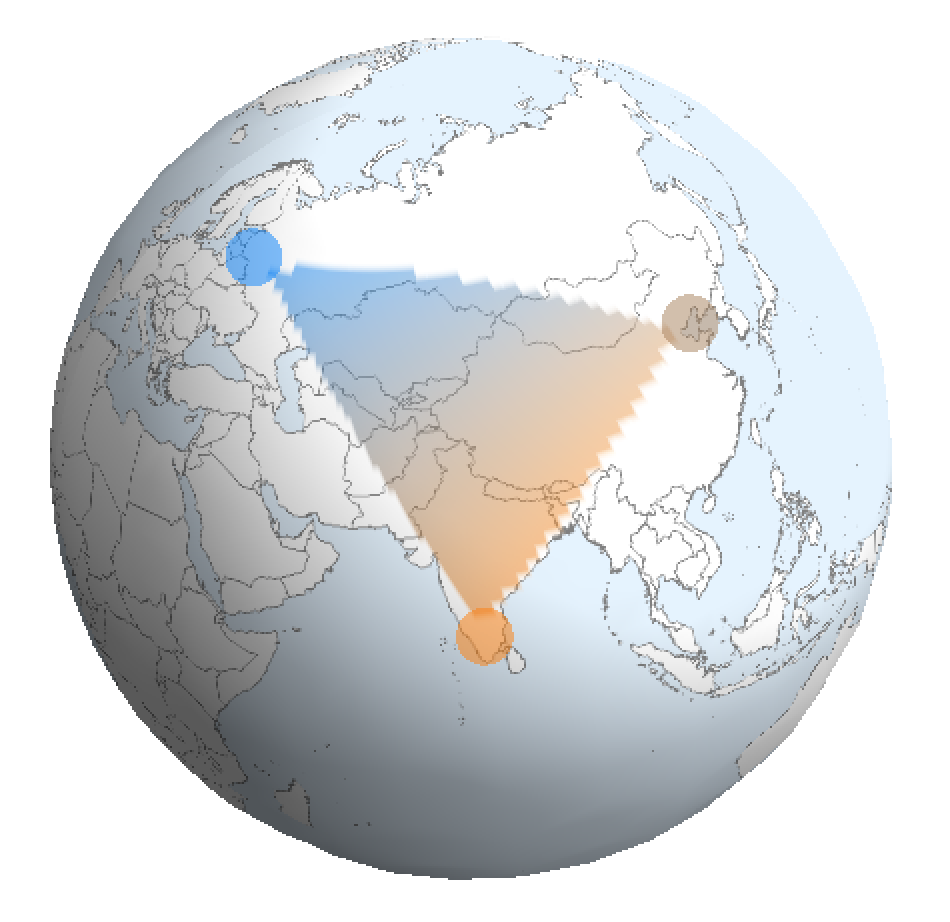From geometry, we can derive an elegant method to do this to a region on a sphere. Using graph theory and enough points, we can extend this to the whole sphere (in the next post).

### The Idea

Here's the ﻿temperature﻿ today in a few cities:

 City Temperature (Celcius) Bangalore 23 Beijing 11 Moscow -12

You want to estimate the temperature in Islamabad using this information - you're a bit crazy like that. You probably already know how to do linear interpolation on the temperature between two points on a one-dimensional line: if you knowand, then you can estimateforby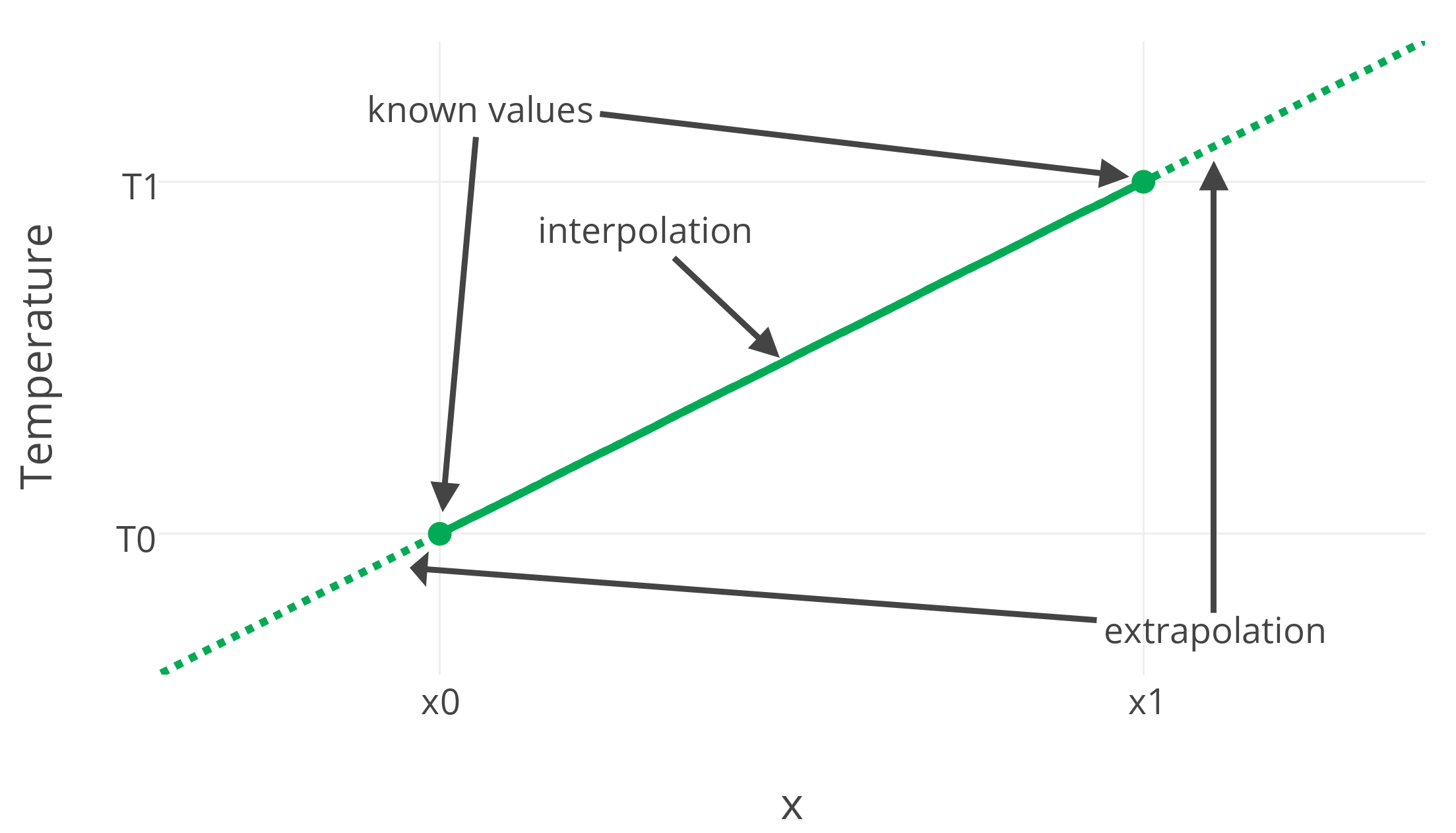But how would you interpolate the temperature between these cities? On a surface like the Earth, the answer isn't so obvious. Not only are we working in two dimensions (latitude and longitude), but the Earth is also curved. I'll show a beautiful way to perform a "linear" interpolation on a sphere.

### Planar Geometry

We can generalize our result on the line to two dimensions. Let's say you know the temperature at pointsand want to estimate it atwithin triangle: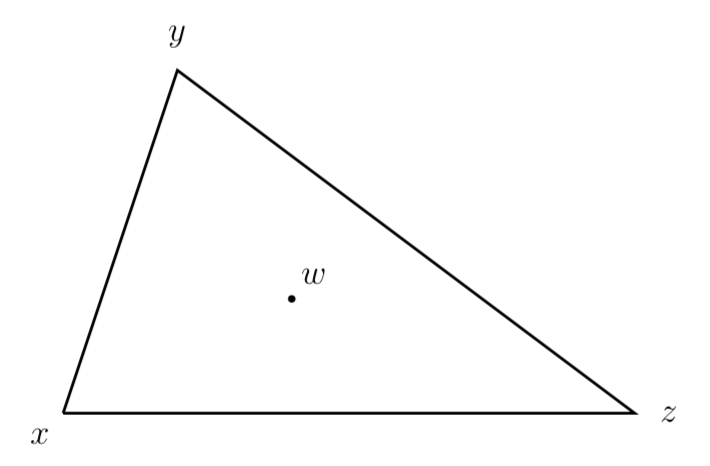We want to estimate the temperature atbyfor some coefficientwith; some mixture of the temperatures. It actually easiest to think of this in vector form, shifting the origin to be whereis: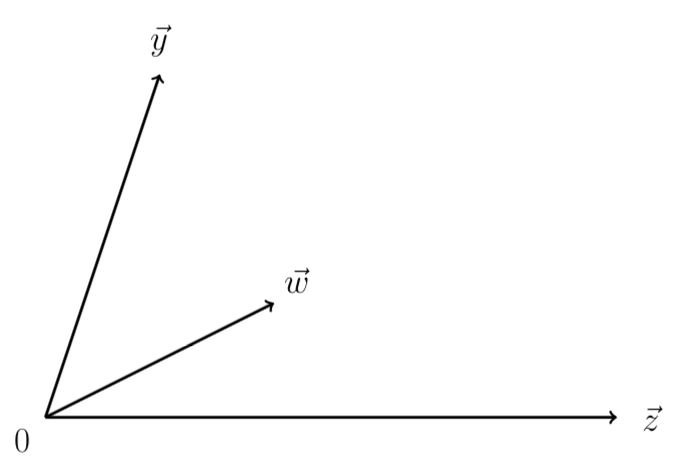Intuitively, the coefficients for our mixture of temperatures should satisfy, leaving. This seems simple enough, but there is a more convenient way to write this in terms of the areas of triangles in the following figure: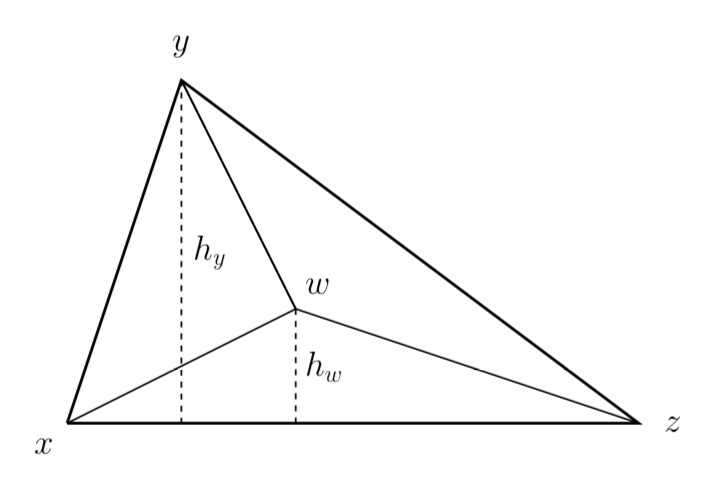Using our vectors from before, the area ofisBecause of the way I have oriented the triangle, it is easy to see that's vertical componentis just(sinceandis entirely horizontal). This givesBy shifting the origin and rotating the triangle, we can apply the same reasoning to obtainThat is, the area of the subtriangle opposite pointis proportional to the coefficentby a ratio equal to the the area of the whole triangle.

### Spherical Geometry

We can actually reduce the problem of interpolating on a sphere to the problem of interpolating on a plane. This is tricky, since there is no such things as a linear function on a sphere - we can only hope for a function which is locally linear. Our previous argument using vectors also breaks down, since following vectors on a sphere in a different order gives a different endpoint. It turns out the best solution is to project everything onto a plane perpendicular to the vector(drawn from the sphere's center) we want to interpolate at: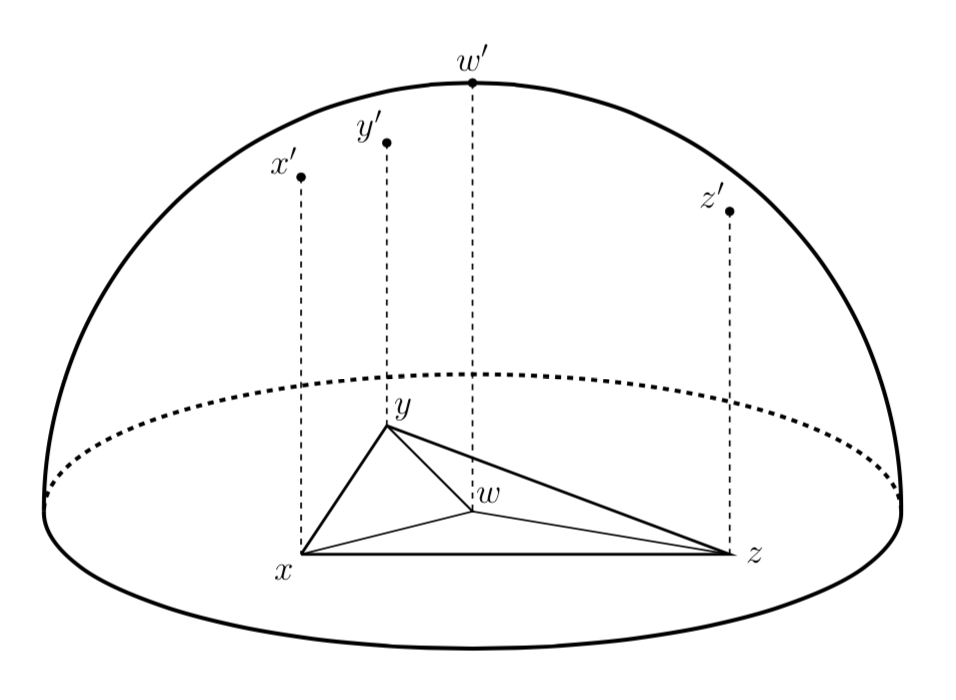Here we have positioned the plane at the base of the hemisphere so that the projected pointis the center of the sphere and the origin. At this juncture, one might despair because actually calculating the projection and evaluating areas from it would be tedious. But fear not: there will be a deus ex machina, and that machina is the determinant. If we take three vectors such as(in 3-space, drawn from) and stuff them into a matrix (as its columns), the determinantof that matrix is very special. Its magnitude iswhereis the volume of the tetrahedron formed byand. Its sign indicates whetherare in counterclockwise order or not, which is a useful fact I will get to in my next blog post.

Anyway, the volume of a tetrahedron is actually extremely useful. You may recall from geometry class thatwhereis the base's area andis the height. From its projection onto the plane, we can chooseand, we getAnd by the same reasoning,In other words, the determinant of the matrix formed by the desired point and two of the known points is proportional to the coefficient for the remaining point by a fixed ratio. So determining the relative proportions of these determinants gives the desired coefficients- a very simple task.

### Result

Using this calculation, we estimate that the temperature in Islamabad is a mixture of 51% that of Bangalore, 14% that of Beijing, and 35% that of Moscow, for a result of 9C. We can also plot the result of this interpolation:The actual temperature there is 15C, which is pretty close (lucky) considering that temperature can vary unpredictably across just a few hundred kilometers. To accurately estimate the temperature using this method, we would need to measure it it many more points; apparently NOAA uses something like 1500 weather stations around the globe. By the time I finished writing this, temperatures had changed slightly, but here's a real heat map of Asia: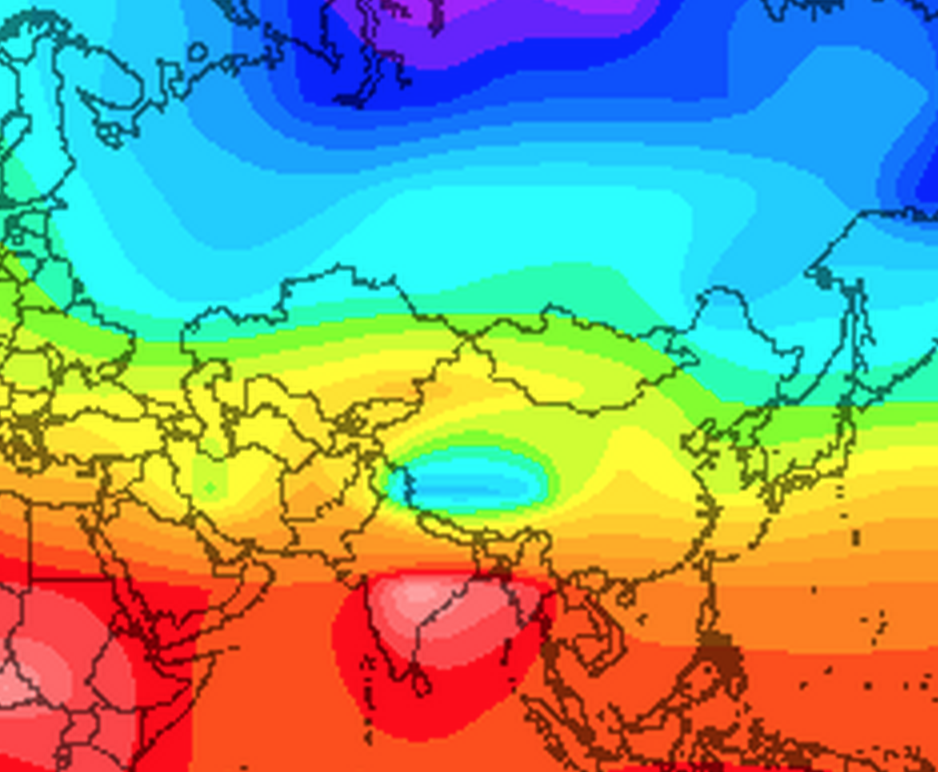I'll show one way to interpolate with very many known points in my next post.